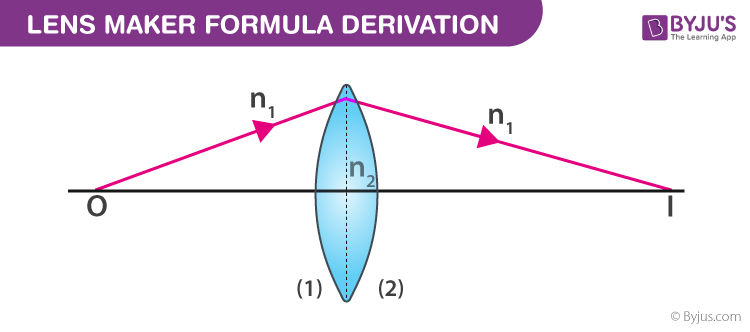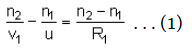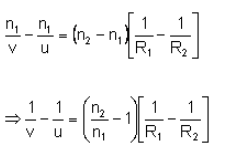# Derivation Of Lens Maker Formula

Lenses of different focal lengths are used for various optical instruments. The focal length of a lens depends upon the refractive index of the material of the lens and the radii of curvatures of the two surfaces. The derivation of lens maker formula is provided here so that aspirants can understand the concept more effectively. Lens manufacturers commonly use the lens maker formula for manufacturing lenses of the desired focal length.

## Lens Maker Formula Derivation### Assumptions

The following assumptions are taken for the derivation of lens maker formula.

• Let us consider the thin lens shown in the image above with 2 refracting surfaces having the radii of curvatures R1 and R2, respectively.
• Let the refractive indices of the surrounding medium and the lens material be n1 and n2, respectively.

Derivation

The complete derivation of lens maker formula is described below. Using the formula for refraction at a single spherical surface we can say that,

For the first surface,For the second surface,Now adding equation (1) and (2),When u = ∞ and v = fBut also,Therefore, we can say that,Where μ is the refractive index of the material.

This is the lens maker formula derivation. Check the limitations of the lens maker’s formula to understand the lens maker formula derivation is a better way.## Limitations of the lens maker’s formula

• The lens should not be thick so that the space between the 2 refracting surfaces can be small.
• The medium used on both sides of the lens should always be the same.

Test your knowledge on Lens maker formula derivation

1. yash magar

nice

2. rohan doke

very useful

3. Soumya Ranjan Meshwa

A well platform for clearing doubt

4. Victoria

This really helped in my revision